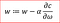# Machine learning : Gradient Descent

For a basic understanding of neural networks

Gradient descent is an iterative machine learning optimization algorithm to reduce the cost function so that we have models that makes accurate predictions.

Cost function(C) or Loss function measures the difference between the actual output and predicted output from the model. Cost function are a convex function.

In neural network our goal is to train the model to have optimized weights(w) to make better prediction.

we get optimized weights using gradient descent.

This is explained best with a classic mountain problem.

In the mountaineering problem we want to reach the lowest point for a mountain and we have zero visibility.

we do not know if we are on the top of the mountain or in the middle of the mountain or very close to the bottom.

Our best option is to check the terrain near us and to identify from where we need to descend to reach the bottom. We need to do this iteratively till there is no more scope to descend and that is when we would have reached the bottom.

we will discuss later in the article what can we do if feel we have reached the bottom(local minimum point) but there is another lowest point for the mountain(global minimum point).

Gradient descent helps us solve the same problem mathematically.

We randomly initialize all the weights for a neural network to a value close to zero but not zero.

we calculate the gradient, ∂c/∂ω which is a partial derivative of cost with respect to weight.

α is learning rate, helps adjust the weights with respect to gradient descentw is the weights for the neurons, α is learning rate, C is the cost and ∂c/∂ω is the gradient

we need to update the weights for all the neurons simultaneously

Learning rate controls how much we should adjust the weights with respect to the loss gradient. Learning rates are randomly initialized.

Lower the value of the learning rate, slower will be the convergence to global minima.

A higher value for learning rate will not allow the gradient descent to converge

Since our goal is to minimize the cost function to find the optimized value for weights, we run multiple iterations with different weights and calculate the cost to arrive at a minimum cost as shown below

It is possible to have two different bottom for the mountain in the same way we can also get local and global minimum points between the cost and weights.

Global minima is minimum point for the entire domain and local minima is a sub optimal point where we get a relatively minimum point but is not the global minimum point as shown below.

How can we avoid local minima and always try and get the optimized weights based on global minima?

so first let’s understand the different type of gradient descent

Different types of Gradient descents are

In batch gradient we use the entire dataset to compute the gradient of the cost function for each iteration of the gradient descent and then update the weights.

Since we use the entire dataset to compute the gradient convergence is slow.

If the dataset is huge and contains millions or billions of data points then it is memory as well as computationally intensive.

• Theoretical analysis of weights and convergence rates are easy to understand

• Perform redundant computation for the same training example for large datasets
• Can be very slow and intractable as large datasets may not fit in the memory
• As we take the entire dataset for computation we can update the weights of the model for the new data

In stochastic gradient descent we use a single datapoint or example to calculate the gradient and update the weights with every iteration.

we first need to shuffle the dataset so that we get a completely randomized dataset. As the dataset is randomized and weights are updated for each single example, update of the weights and the cost function will be noisy jumping all over the place as shown below

Random sample helps to arrive at a global minima and avoids getting stuck at a local minima.

Learning is much faster and convergence is quick for a very large dataset.

• Learning is much faster than batch gradient descent
• Redundancy is computation is removed as we take one training sample at a time for computation
• Weights can be updated on the fly for the new data samples as we take one training sample at a time for computation

• As we frequently update weights, Cost function fluctuates heavily

Mini-batch gradient is a variation of stochastic gradient descent where instead of single training example, mini-batch of samples is used.

Mini batch gradient descent is widely used and converges faster and is more stable.

Batch size can vary depending on the dataset.

As we take a batch with different samples,it reduces the noise which is variance of the weight updates and that helps to have a more stable converge faster.

• Reduces variance of the parameter update and hence lead to stable convergence
• Speeds the learning
• Helpful to estimate the approximate location of the actual minimum